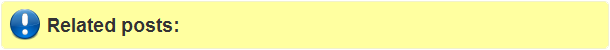## Friday, August 12, 2016

### Random Forest Regression, Negative Variance Explained mechanism

Jeffery Evans, Senior Landscape Ecologist, The Nature Conservancy, Global Lands Science Team, Affiliate Assistant Professor, Zoology & Physiology, University of Wyoming explains a negative percent variance explained in a random forest regression in hilarious way -

I have recently been asked the question: “why do I receive a negative percent variance explained in a random forest regression”. Besides the obvious answer “because your model is crap” I thought that I would explain the mechanism at work here so the assumption is not that randomForests is producing erroneous results. For poorly supported models it is, in fact, possible to receive a negative percent variance explained.

Generally, explained variance (R²) is defined as:

R² = 1 - sum((ŷ-mean(y))²) / sum((mean(y)-y)²)

However, as indicated by Breiman (2001) and the R randomForest documentation the (regression only) “pseudo R-squared” is derived as:

R² = 1 – (mean squared error) / var(y)

Which, mathematically can produce negative values. A simple interpretation of a negative R² (rsq), is that you are better off predicting any given sample as equal to overall estimated mean, indicating very poor model performance.

Here is a simple example of a random forests regression model producing a negative R2 with comparison to the Pearson and Spearman correlation coefficients.

##################################
library(randomForest)
obs = 500
vars = 100
x = replicate(vars,factor(sample(1:5,obs,replace=TRUE)))
y = rnorm(obs)
( rf.regression = randomForest(x, y) )
## Variance explained
cat("% Var explained: \n", 100 * (1-sum((rf.regression \$y- rf.regression \$pred   )^2) /
sum((rf.regression \$y-mean(rf.regression \$y))^2)))
### Plot observed vs. predected
plot(rf.regression \$y, rf.regression \$predicted, pch=20)

## Pearson correlation R²
cat("% Pearson correlation: \n ", 100*  cor(rf.regression \$y, rf.regression \$predicted)^2)
## Spearman correlation R²
cat("% Spearman correlation \n ", 100 * cor(rf.regression \$y, rf.regression \$predicted, method="s")^2)

###################################### 1 comment :

1.